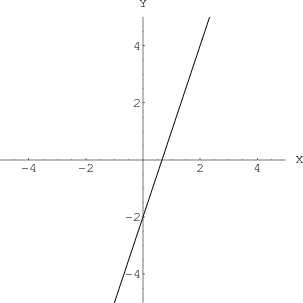# Math Insight

### Image: A line in two dimensionsThe graph of the equation $y=3x-2$ is a line, if it is an equation in two dimensions.

Image file: line_in_2D.png